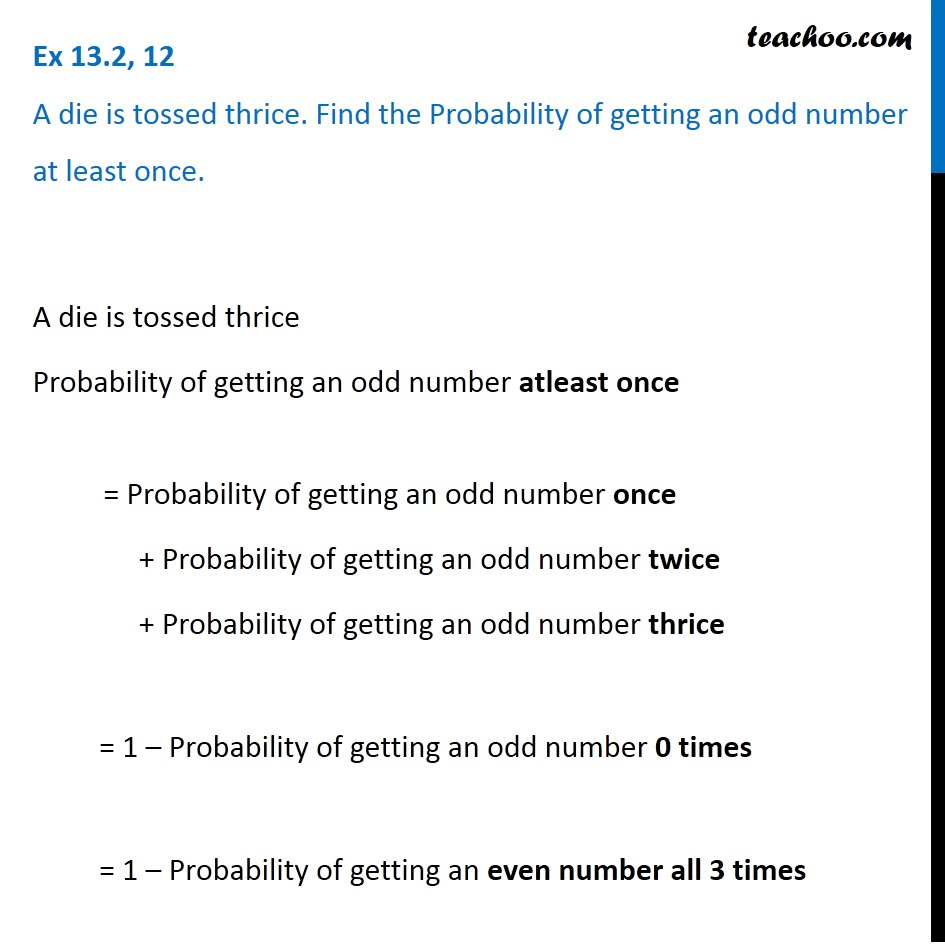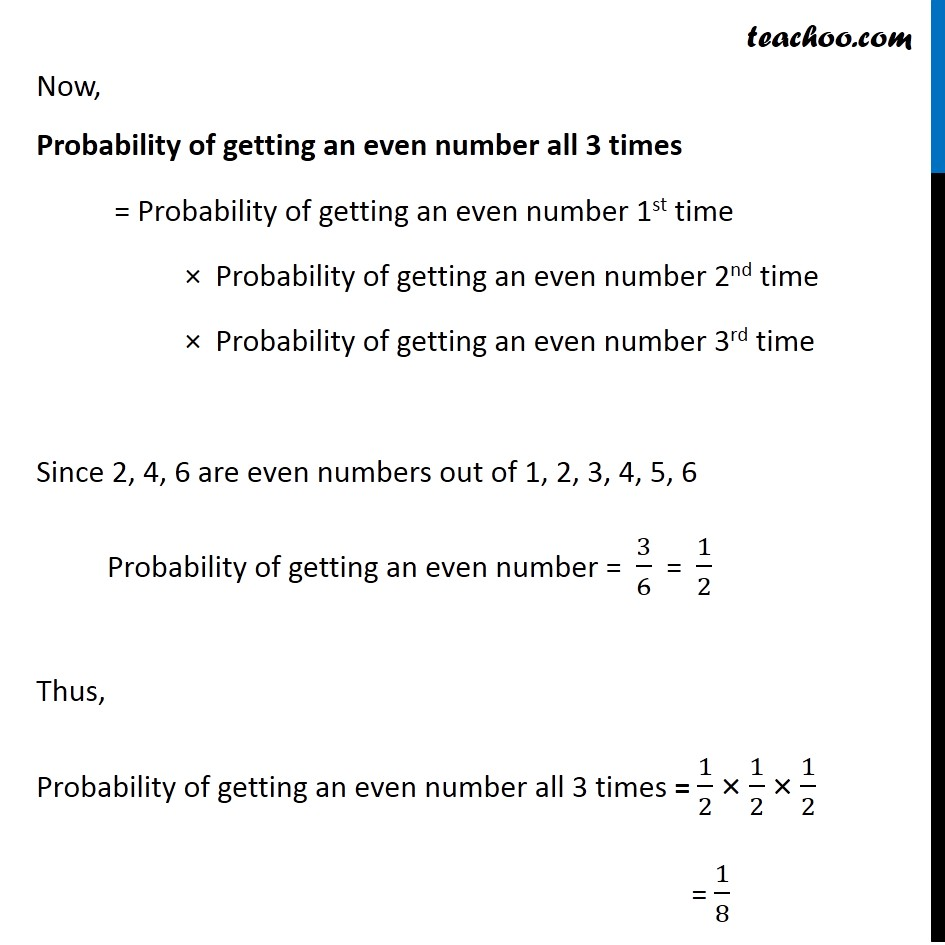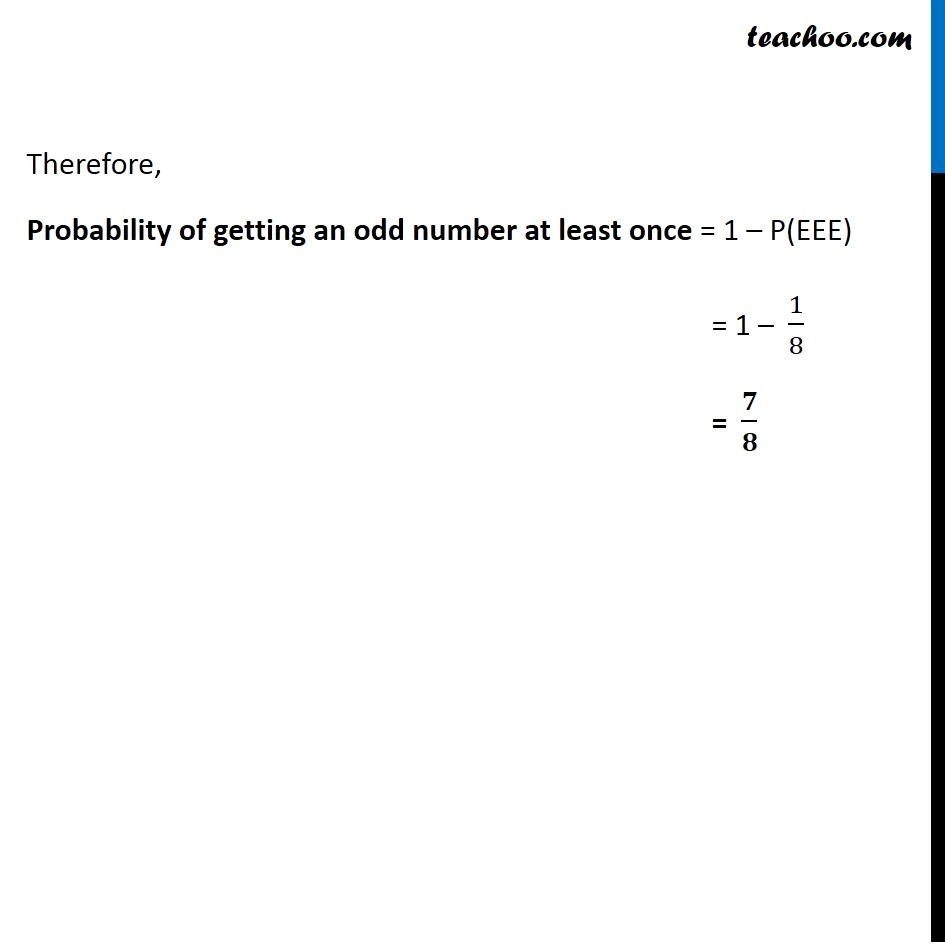Basic Probability

Chapter 13 Class 12 Probability
Concept wiseIntroducing your new favourite teacher - Teachoo Black, at only ₹83 per month

### Transcript

Ex 13.2, 12 A die is tossed thrice. Find the Probability of getting an odd number at least once.A die is tossed thrice Probability of getting an odd number atleast once = Probability of getting an odd number once + Probability of getting an odd number twice + Probability of getting an odd number thrice = 1 – Probability of getting an odd number 0 times = 1 – Probability of getting an even number all 3 times Now, Probability of getting an even number all 3 times = Probability of getting an even number 1st time × Probability of getting an even number 2nd time × Probability of getting an even number 3rd time Since 2, 4, 6 are even numbers out of 1, 2, 3, 4, 5, 6 Probability of getting an even number = 3/6 = 1/2 Thus, Probability of getting an even number all 3 times = 1/2 × 1/2 × 1/2 = 1/8 Therefore, Probability of getting an odd number at least once = 1 – P(EEE) = 1 – 1/8 = 𝟕/𝟖